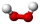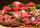# Peroxide

How many ml 30% of peroxide (H2O2) should be poured into 100ml H2O to give a 20% solution?

Result

x =  200 ml

#### Solution:Leave us a comment of example and its solution (i.e. if it is still somewhat unclear...):

Showing 0 comments:Be the first to comment!#### To solve this example are needed these knowledge from mathematics:

Looking for a statistical calculator? Our percentage calculator will help you quickly calculate various typical tasks with percentages. Do you have a linear equation or system of equations and looking for its solution? Or do you have quadratic equation? Tip: Our volume units converter will help you with converion of volume units.

## Next similar examples:

1. CloggingHow much distilled water must the pharmacist add to 30g of a 30% hydrogen peroxide solution to obtain a 3% solution to clogging?
2. SaltSea water containing 5% salt. How many kg of fresh water necessary to pour into 40 kg of sea water so that the salinity fell to 2%?
3. Solutions, mixturesHow many liters of 70% solution we must add to 5 liters of 30% solution to give us a 60% solution?
4. Acid solutionBy adding 250 grams of a 96% solution of sulfuric acid to its 3% solution its initial concentration was changed to 25%. How many grams of 3% of the acid were used for dilution?
5. CacaoCacao contains 34% filling. How many grams of filling are in 130 g cacao.
6. Acid evaporationHow many kilograms of water do we have to evaporate from 100 kg of 32% acid to make it 80% concentration?
7. MushroomsMushrooms lose 90% by weight drying. How many fresh mushrooms are needed for 5 kg of dried mushrooms?
8. GirlsThe children's competition was attended by 63 girls, which is 30% of all children's participants. How many children attended this competition?
9. Sales offThe price has decreased by 20%. How many percents do I have to raise the new price to be the same as before the cut?
10. Frameworks is badCalculate how many percent will increase the length of an HTML document, if any ASCII character unnecessarily encoded as hexadecimal HTML entity composed of six characters (ampersand, grid #, x, two hex digits and the semicolon). Ie. space as: &#x20;
11. Sale discountThe product was discounted so that eight products at a new price cost just as five products at an old price. How many percents is the new price lower than the old price?
12. The ballThe ball was discounted by 10 percent and then again by 30 percent. How many percent of the original price is now?
13. Meat losesMeat loses 18% of its weight by smoking. How much raw meat butcher used to manufacture 35 kilos of smoked?
14. Perctentages35% of what number is 35?
15. Fifth of the numberThe fifth of the number is by 24 less than that number. What is the number?
16. CompetitorsIn the first round of slalom fell 15% of all competitors and in the second round another 10 racers. Together, 40% of all competitors fell. What was the total number of competitors?
17. Profit gainIf 5% more is gained by selling an article for Rs. 350 than by selling it for Rs. 340, the cost of the article is: